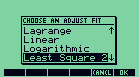Interpolation is a program that calculates many kinds of interpolation. It calculates liner fit, power fit, logarithmic fit, lagrange fit, least square of second degree, least squares of third degree and also shows examples of plots of these functions.Screenshot of Interpolation